Sponsored Link •writing a number variable to a file

4 replies on 1 page. Most recent reply: Dec 12, 2018 2:07 AM by Vijay Khatri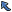Back to Topic List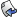Reply to this Topic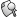Search Forum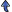Threaded View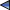Previous Topic Next Topic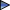Flat View: This topic has 4 replies on 1 page
 not telling Posts: 2 Nickname: ulrich200 Registered: Oct, 2008
writing a number variable to a file Posted: Oct 18, 2008 6:04 PMReply
 AdvertisementHi I'm new to python and I'm trying to make a program to keep track of my money, but when it tries to write the number i get this message:

`TypeError: unsupported operand type(s) for +: 'float' and 'str'`

`moneyRead = open("my_money.txt", "r")moneyWrite = open("my_money.txt", "w")choice = 0choice2 = 0loop = 1value = 0value1 = 0value2 = 0value3 = 0print " "print "Welcome!"print " "print "To view the amount of money you have, type: 1"print "To set the amount of money you have, type: 2"print "To add or subtract to the amount of money you have, type: 3"print "to quit, type: 4"print " "while loop == 1:    choice = input("What do you want to do?: ")    print " "    if choice == 1:        print moneyRead.readline()    if choice == 2:        value = input("How much money do you have?: ")        moneyWrite.write(value)    if choice == 3:        print "The current saved amount is " + moneyRead.readline()        print " "        print "To add to this amount, type: 1"        print "To subtract from this amount, type: 2"        choice2 = input("What do you want to do?: ")            if choice2 == 1:            value1 = moneyRead.readline()            value2 = input("how much do you want to add?: ")            value3 = value1 + value2            moneyWrite.write(value3)        if choice2 == 2:            value1 = moneyRead.readline()            value2 = input("how much do you want to subtract?: ")            value3 = value1 - value2            moneyWrite.write(value3 )        if choice == 4:        loop = 0`

 not telling Posts: 2 Nickname: ulrich200 Registered: Oct, 2008
Re: writing a number variable to a file Posted: Oct 18, 2008 6:12 PMReply
I messed up on the error message.

here's the real error message:

`TypeError: argument 1 must be string or read-only character buffer, not float`

 ndoe ganteng Posts: 3 Nickname: ndoe Registered: Oct, 2008
Re: writing a number variable to a file Posted: Nov 3, 2008 9:22 AMReply
how to make a paragraph from file to file

like this e.g data.txt

i
want
eat
some
pizza
with
cappucino
in
kuta
bali

to this data2.txt

i want eat some pizza with cappucino in kuta bali

but without exchange the code i free change data.txt

e.g

i
want
eat
some
pizza
with
cappucino
in
kuta
bali
late
night

i want eat some pizza with cappucino in kuta bali late night

 captain eddy Posts: 4 Nickname: hemsbond Registered: Oct, 2010
Re: writing a number variable to a file Posted: Nov 8, 2010 1:04 AMReply
`moneyRead = open("my_money.txt", "r")moneyWrite = open("my_money.txt", "w")choice = 0choice2 = 0loop = 1value = 0value1 = 0value2 = 0value3 = 0print " "print "Welcome!"print " "print "To view the amount of money you have, type: 1"print "To set the amount of money you have, type: 2"print "To add or subtract to the amount of money you have, type: 3"print "to quit, type: 4"print " "while loop == 1:    choice = input("What do you want to do?: ")    print " "    if choice == 1:        print moneyRead.readline()    if choice == 2:        value = raw_input("How much money do you have?: ")        moneyWrite.write(value)    if choice == 3:        print "The current saved amount is " + moneyRead.readline()        print " "        print "To add to this amount, type: 1"        print "To subtract from this amount, type: 2"        choice2 = input("What do you want to do?: ")            if choice2 == 1:            value1 = moneyRead.readline()            value2 = raw_input("how much do you want to add?: ")            value3 = value1 + value2            moneyWrite.write(value3)        if choice2 == 2:            value1 = moneyRead.readline()            value2 = raw_input("how much do you want to subtract?: ")            value3 = value1 + value2            moneyWrite.write(value3 )        if choice == 4:        loop = 0`

 Vijay Khatri Posts: 2 Nickname: vijay1994 Registered: Dec, 2018
Re: writing a number variable to a file Posted: Dec 12, 2018 2:07 AMReply
Any of these should work

outf.write("%s" % num)

outf.write(str(num))

print >> outf, numPrevious Topic Next TopicWeb Artima.com